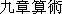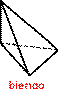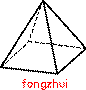Previous page || Next page || First page || References

Click on any figure to see it enlarged in a separate window.

## 3. Rectilinear solids treated by Liu Hui

Chapter 5 of the Jiuzhang suanshuis ostensibly concerned with earthworks and the amount of labour needed to build them. The only parts of the chapter which are especially interesting mathematically, however, are those which give algorithms for calculating the volumes of solids. For each of these algorithms, Liu Huigives a derivation. The order of his derivations is dictated by the order in which the solids are discussed in the Jiuzhang suanshu, but the derivations can be placed in a logical order so that each depends only-upon those which precede it; Liu Hui is never guilty of circular reasoning.

In the present article I summarise one of Liu Hui's derivations (Section 4 below) and translate one "Section 6). Below I describe briefly each of the rectilinear solids treated by Liu Hui.

The volume of a box, or rectangular parallelopiped, the product of its three dimensions, is implicitly assumed, and Liu Hui makes no attempt to explain it.A qiandu(lit., "moat-wall") is a right prism with right-triangular base [Jiuzhang, 166].A yangma(lit., "male horse," but originally an architectural term; see Section 6.1 below) is a pyramid with rectangular base and with one lateral edge perpendicular to the base [Jiuzhang, 166-168]. Liu Hui's derivation of its volume is translated in Section 6 below.A bienao(lit., "turtle's shoulder-joint") is a pyramid with right-triangular base and with one lateral edge perpendicular to the base. The perpendicular edge is not to be at the right-angled vertex of the base [Jiuzhang, 168]. Liu Hui's derivation of its volume is an integral part of his derivation of the volume of a yangma; see Section 6.In one of his derivations (that of the volume of a xianchu, see below), Liu Hui uses a pyramid with right-triangular base and with the lateral edge at the right-angled vertex of the base perpendicular to the base. This he unfortunately also calls a bienao; but he is aware that his derivation of the volume of a bienao does not apply to this object, and he gives a separate derivation of its volume [Jiuzhang, 168-169].A fangzhui(lit., "square awl") is a right pyramid with square base [Jiuzhang, 165}.A chumeng(lit., "fodder loft") is a right wedge with rectangular base [Jiuzhang, 169-170].A chutong(lit., "fodder boy", probably originally some sort of agricultural implement or receptacle) is a truncated right pyramid with rectangular base [Jiuzhang, 170]. This object is also referred to by the terms panchi(lit. "winding moat"), quchi("crooked moat"), and minggu("dark valley"). [Jiuzhang, 170-173]. The etymologies of these terms are obscure.A fangting(lit., "square pavilion") is a truncated right pyramid with square base [Jiuzhang, 164]. Obviously the fangting is a special case of the chutong; Liu Hui does not use this fact, but treats the two independently. Liu Hui's derivation of the volume of a fangting is summarised in Section 4 below.A xianchu(lit., "drain"?) is a wedge with trapezoidal base and with one lateral face perpendicular to the base [Jiuzhang, 168-169]. In treating the volume of a xianchu, Liu Hui runs into a mathematical situation which is too complex for his methods and conceptual framework; the result is a very obscure text. A very tentative translation of this derivation has been given in [Wagner 1975].
It can be seen from the above that Liu Hui deals only with some very specific geometrical objects; there is no attempt to generalise, even though this would in many cases be rather simple. For example, his normal method could be used to derive the volume of a general pyramid as one-third of the product of the height and the area of the base. This failure to generalise can in part be explained by reference to the nature of Liu Hui's book: it is a commentary on an existing classical work, and therefore its only concern is to explain the statements made in it, not to extend it. Furthermore, Liu Hui's purpose is probably primarily pedagogical. A student who has mastered his methods can easily handle a great variety of geometrical figures as the need arises.

Another feature which will be apparent in the above discussion is that most of the terms for these solids refer to practical objects. This should not lead to the immediate conclusion that ancient Chinese mathematics was only concerned with practical matters: certainly it was more practically oriented than Greek mathematics, but the use of terms like "fodder loft" to denote geometrical figures is not really different from our use of terms like "pyramid."

In an unpublished thesis [Wagner 1975] I have translated Liu Hui's derivations of the volumes of the fangting, yangma, and xianchu.

The derivation would begin with the fact that the formula holds for any pyramid with triangular base and with one lateral edge perpendicular to the base. (Such a pyramid is either the sum or the difference of two bienao.) Next the formula could be derived for the case of a pyramid whose base is a convex n-sided polygon and whose altitude intersects the base. (Such a pyramid can be divided into n pyramids of the type considered above.) The general case would be considerably more complex, but not absolutely beyond Liu Hui's methods.

Previous page || Next page || First page || References